##### Organic Chemistry I For Dummies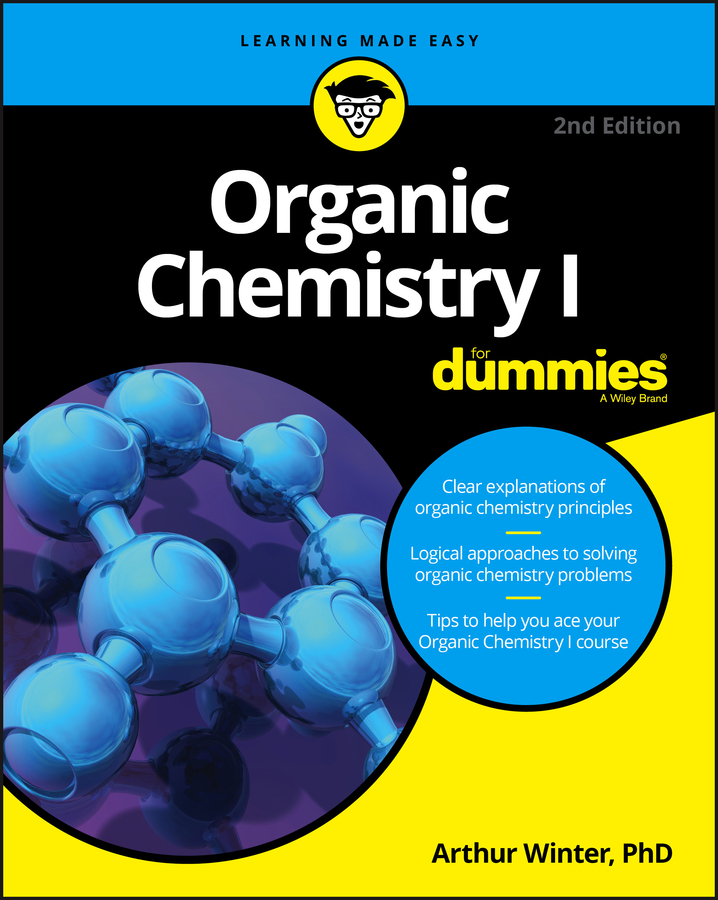Redox equations are often so complex that fiddling with coefficients to balance chemical equations doesn’t always work well. Chemists have developed an alternative method (in addition to the oxidation number method) that is called the ion-electron (half-reaction) method.

In the ion-electron method, the unbalanced redox equation is converted to the ionic equation and then broken down into two half-reactions — oxidation and reduction. Each of these half-reactions is balanced separately and then combined to give the balanced ionic equation.

Finally, the spectator ions are put into the balanced ionic equation, converting the reaction back to the molecular form. It’s important to follow the steps precisely and in the order listed. Otherwise, you may not be successful in balancing redox equations.

The example below shows you how to use the ion-electron method to balance this redox equation: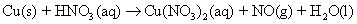1. Convert the unbalanced redox reaction to the ionic form.

In this reaction, you show the nitric acid in the ionic form, because it’s a strong acid. Copper (II) nitrate is soluble — indicated by (aq) — so it’s shown in its ionic form. Because NO(g) and water are molecular compounds, they remain shown in the molecular form: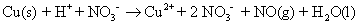2. If necessary, assign oxidation numbers and then write two half-reactions (oxidation and reduction) showing the chemical species that have had their oxidation numbers changed.

In some cases, it’s easy to tell what has been oxidized and reduced; but in other cases, it isn’t as easy. Start by going through the example reaction and assigning oxidation numbers. You can then use the chemical species that have had their oxidation numbers changed to write your unbalanced half-reactions: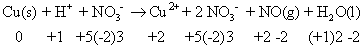Copper changed its oxidation number (from 0 to 2) and so has nitrogen (from –2 to +2). Your unbalanced half-reactions are: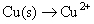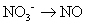3. Balance all atoms, with the exception of oxygen and hydrogen.

It’s a good idea to wait until the end to balance hydrogen and oxygen atoms, so always balance the other atoms first. You can balance them by fiddling with the coefficients. (You can’t change subscripts; you can only add coefficients.) However, in this particular case, both the copper and nitrogen atoms already balance, with one each on both sides:4. Balance the oxygen atoms.

How you balance these atoms depends on whether you’re dealing with acid or basic solutions:

• In acid solutions, take the number of oxygen atoms needed and add that same number of water molecules to the side that needs oxygen.

• In basic solutions, add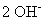to the side that needs oxygen for every oxygen atom that is needed. Then, to the other side of the equation, add half as many water molecules as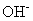anions used.

The example equation is in acidic conditions. There’s nothing to do on the half-reaction involving the copper, because there are no oxygen atoms present. But you do need to balance the oxygen atoms in the second half-reaction: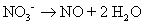5. Balance the hydrogen atoms.

Again, how you balance these atoms depends on whether you’re dealing with acid or basic solutions:

• In acid solutions, take the number of hydrogen atoms needed and add that same number of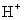to the side that needs hydrogen.

• In basic solutions, add one water molecule to the side that needs hydrogen for every hydrogen atom that’s needed. Then, to the other side of the equation, add as manyanions as water molecules used.

The example equation is in acidic conditions. You need to balance the hydrogen atoms in the second half-reaction: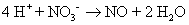6. Balance the ionic charge on each half-reaction by adding electrons.

The electrons should end up on opposite sides of the equation in the two half-reactions. Remember that you’re using ionic charge, not oxidation numbers.

Oxidation: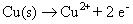Reduction: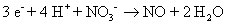7. Balance electron loss with electron gain between the two half-reactions.

The electrons that are lost in the oxidation half-reaction are the same electrons that are gained in the reduction half-reaction. The number of electrons lost and gained must be the same. But Step 6 shows a loss of 2 electrons and a gain of 3.

So you must adjust the numbers using appropriate multipliers for both half-reactions. In this case, you have to find the lowest common denominator between 2 and 3. It’s 6, so multiply the first half-reaction by 3 and the second half-reaction by 2.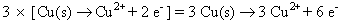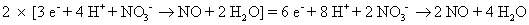8. Add the two half-reactions together and cancel anything common to both sides.

The electrons should always cancel (the number of electrons should be the same on both sides).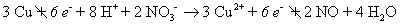9. Convert the equation back to the molecular form by adding the spectator ions.

If it’s necessary to add spectator ions to one side of the equation, add the same number to the other side of the equation.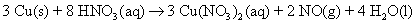10. Check to make sure that all the atoms are balanced, all the charges are balanced (if working with an ionic equation at the beginning), and all the coefficients are in the lowest whole-number ratio.

Reactions that take place in base are just as easy, as long as you follow the rules.